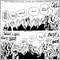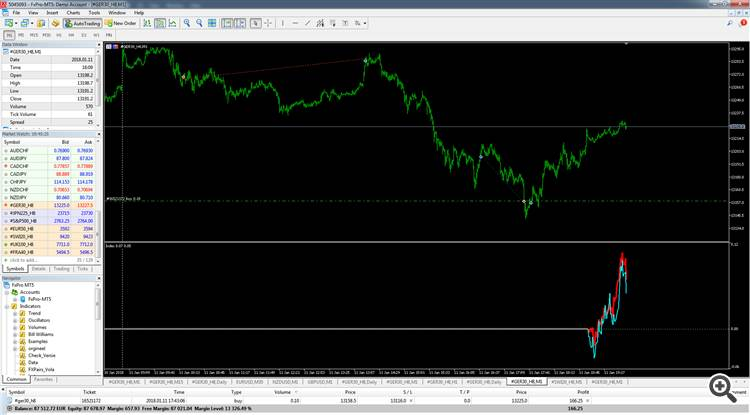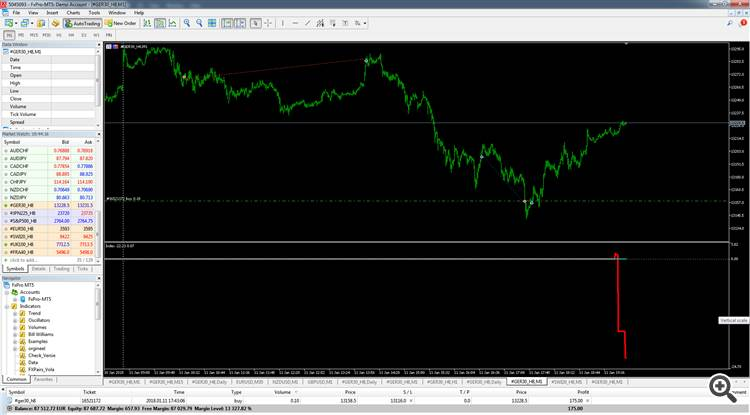Need help for calculating relative price Index basket707

Hi all.

I'm trying to built a 'relative price index' based on a number of major world Indexes: S&P500, DAX30, EUR50, CAC40, SWISS20, JPN225 and UK100.

My problem is that the calculated output is not reliable. Sometimes it works but often the indicator skips data and the average price is not correct. (The CopyClose() function is not working properly)

I think the reason is the excessive use of the CopyClose() function is the blame but i'm not sure. Now the function is called 7 times for each M1 bar foreach symbol in a double loop function, see the code.

Can anybody explain why this is not working correct.//+------------------------------------------------------------------+
//|                                              Index_Variables.mqh |
//|                        Copyright 2017, MetaQuotes Software Corp. |
//|                                              http://www.mql5.com |
//+------------------------------------------------------------------+
#property copyright "Copyright 2017, MetaQuotes Software Corp."
#property strict

#define SIZE1 50 // Aantal Bars in het verleden
#define SIZE2 7 // Totaal aantal beschikbare Indexen
#define SIZE3 2 // Diepte van de 3 dimensionale array.
#define SIZE4 2 // Gemiddelde waarde en DAX index waarde.

struct Str_Data
{

int      nLowest_Bar_Number_Value; // Het laagste aantal beschikbare BARS.

datetime adTime_Main_Variables[SIZE1]; // Array met de tijd/datum data van de referentie Index (DAX).

datetime adTimeData[SIZE1][SIZE2]; // Multidimensionale array met de datums van aller BARS van de verschillende Indexen.

string sSymbol1;
string sSymbol2;
string sSymbol3;
string sSymbol4;
string sSymbol5;
string sSymbol6;
string sSymbol7;

int    nIndexHistory_Bar_Counter; // Indexnummer voor de indexering van het aantal bars in het verleden.
int    nTime_Period; // Het aantal bars in het verleden waarover de indicator berekend wordt.
int    nTotal_Array_Length; // De totale lengte van de data array.
int    nBarCounter;  // Aflopende teller van het aantal BARS in het verleden.
int    nIndex_i;

int      anIndexNumber[SIZE2][SIZE3];     // Indexnummer voor het opzoeken van de juiste datum/prijs en het aantal beschikbare BARS.
datetime atTimeDateData[SIZE1+841];

double adData[SIZE1][SIZE2]; // 2 dimensionale data array
double adBigData[SIZE1+841][SIZE2][SIZE3]; // 3 dimensionale data array.
double adAveragePrice[SIZE1+841][SIZE4]; // De gemiddelde prijs van alle indexen.
double adStart_Refprice[SIZE2]; // De startwaarde van de referentie prijs per Index.

string asSymbols[SIZE2];
int    nSymbol_Index_Number;

//-----------------------------
datetime dDay_Period;
datetime dM1_Period;

datetime adTimeDate_Data[];  // Array met de tijd en datum van de hoofd (DAX) index.
};

void Reset_Data(Str_Data &var)
{

}

void Initialize_Data(Str_Data &var)
{
var.sSymbol1 = "#GER30_H8";
var.sSymbol2 = "#S&P500_H8";
var.sSymbol3 = "#EUR50_H8";
var.sSymbol4 = "#JPN225_H8";
var.sSymbol5 = "#UK100_H8";
var.sSymbol6 = "#SWI20_H8";
var.sSymbol7 = "#FRA40_H8";

var.nLowest_Bar_Number_Value  = 0;
var.nTime_Period = SIZE1;
var.nBarCounter  = SIZE1;
var.nIndexHistory_Bar_Counter = 0;

var.dDay_Period = PERIOD_D1;
var.dM1_Period  = PERIOD_M1;

var.nTotal_Array_Length = var.nTime_Period + 901; // Lengte van de indicator plus extra marge voor de realtime tiks van de huidige dag.

var.asSymbols = var.sSymbol1; var.asSymbols = var.sSymbol2; var.asSymbols = var.sSymbol3; var.asSymbols = var.sSymbol4; var.asSymbols = var.sSymbol5; var.asSymbols = var.sSymbol6; var.asSymbols = var.sSymbol7;

for(int i = 0; i < ArrayRange(var.adTimeData,0); i++)
{
for(int x = 0; x < ArrayRange(var.adTimeData,1); x++)
{
}
}

for(int i = 0; i < ArrayRange(var.adBigData,0); i++)
{
for(int x = 0; x < ArrayRange(var.adBigData,1); x++)
{
for(int z = 0; z < ArrayRange(var.adBigData,2); z++)
{
}

}
}
}

void fCopy_TimeDate(Str_Data &var)
{
int      nCounter = 0;     // Teller voor het aantal pogingen om de data te laden.
bool     bFail    = false; // Bevestiging boolean indien het ophalen van de data mislukt.
datetime atDataArray[];    // array voor de data van de tijd/datum.

do {
// Kopieer de tijd en datum gegevens van de DAX naar de array.
if(CopyTime(Symbol(), PERIOD_M1, 1, var.nBarCounter, atDataArray) == -1) {bFail = true;};
nCounter++;

if(nCounter == 50) {Alert("fout tijdens het ophalen van de data bij Index: ", Symbol());}
}
while(bFail == true && nCounter <= 50);

// Indien de indicator in het heden draait, moet hij de data van de laatste 4 bars ophalen.
int nNumberOfBars = 1;
if(var.nBarCounter <= 1) {nNumberOfBars = 4;}

// Loop voor het ophalen van de tijd/datum van de hoofdindex (DAX).
for(int a = 0; a < var.nBarCounter; a++)
{
var.adTimeDate_Data[var.nIndexHistory_Bar_Counter] = atDataArray[a]; // Kopieer de data naar de datum array.

for(int z = 0; z < nNumberOfBars; z++)
{
int nSum_Counter  = 0; // Som van het aantal geldige index signalen.

// Loop voor het ophalen van de data van de verschillende Indexen.
for(int x = 0; x < ArraySize(var.asSymbols); x++)
{
fIndex_Relative_Price_Data(var, var.asSymbols[x], x, z, nSum_Counter, nNumberOfBars);  // Bevestiging boolean dat er een geldige datum aanwezig is.
}

// Berekening van het % gemiddelde.
fAverage_Index_Price_Data(var, nNumberOfBars, z);
}

var.nIndexHistory_Bar_Counter++; // Verhoog de indexteller.
}
}

void fIndex_Relative_Price_Data(Str_Data &var,
string sSymbol,
int    x,             // Indexering van het Symbool Type nr.
int    z,             // Aantal keren dat de data moet worden opgehaald. normaal is het 1 keer maar bij de meest recente BARS moet het model 4 BARS terug in het verleden.
int    nSum_Counter,  // Het aantal geldige indexen waarvan de data beschikbaar is.
int    nNumberOfBars) // De verschuiving van het aantal BARS in het verleden.
{

// Berekening van het aantal BARS in het verleden.
int nIndex_nr = var.nIndexHistory_Bar_Counter - (nNumberOfBars - (z + 1));

double dClose_Data;

int    nCounter = 0;
bool   bFail = false;

do {
if(CopyClose(sSymbol, PERIOD_M1, var.adTimeDate_Data[nIndex_nr], 1, dClose_Data) == -1) {bFail = true;};

nCounter++;

if(nCounter == 50) {Alert("fout tijdens het ophalen van de data bij Index: ", sSymbol);}
if(bFail == false && dClose_Data > 0) {nSum_Counter++;}
}
while(bFail == true && nCounter <= 50);

// % afwijking berekenen van de huidige gemiddelde prijs tov de eerste waarde in de array.
}

void fAverage_Index_Price_Data(Str_Data &var,
int    nNumberOfBars, // De verschuiving van het aantal BARS in het verleden.
int    z)
{

// Berekening van het aantal BARS in het verleden.
int nIndex_nr = var.nIndexHistory_Bar_Counter - (nNumberOfBars - (z + 1));

double dSom_Value = 0; // De Somwaarde van de gemiddelde prijzen.
int    nCounter   = 0; // Het aantal geldig prijzen.

for(int x = 0; x < ArraySize(var.asSymbols); x++)
{
// De waarde is groter dan 0.
{
nCounter++;
dSom_Value = dSom_Value + var.adBigData[nIndex_nr][x];
}

// Controleer of het symbool gelijk is aan de DAX.
if(var.asSymbols[x] == var.sSymbol1)
{
}
}

// Bereken het gemiddelde.
if(nCounter > 0)
{
}
}

void fNumber_Of_Bars_Check(Str_Data &var)
{

var.nLowest_Bar_Number_Value = 0;

for(int i = 0; i < ArraySize(var.asSymbols); i++)
{

var.anIndexNumber[i] = Bars(var.asSymbols[i], PERIOD_M1);

if(var.nLowest_Bar_Number_Value <= 0 || var.anIndexNumber[i] < var.nLowest_Bar_Number_Value) {var.nLowest_Bar_Number_Value = var.anIndexNumber[i];}
Alert(var.asSymbols[i], "  ", var.anIndexNumber[i], "  ", var.nLowest_Bar_Number_Value);
}

}39023

Moved to right section.

That's a lot of code to debug...not sure you will get an answer.19843

Snelle Moda: I think the reason is the excessive use of the CopyClose() function is the blame but i'm not sure. Now the function is called 7 times for each M1 bar foreach symbol in a double loop function, see the code.
if(CopyClose(sSymbol, PERIOD_M1, var.adTimeDate_Data[nIndex_nr], 1, dClose_Data) == -1) {bFail = true;};

Can anybody explain why this is not working correct.

On MT4: Unless the current chart is the specific pair/TF referenced, you must handle 4066/4073 errors.
Download history in MQL4 EA - MQL4 and MetaTrader 4 - MQL4 programming forum

On MT5: Unless the chart is that specific pair/TF, you must Synchronize the terminal Data from the Server.
Timeseries and Indicators Access /  Data Access - Reference on algorithmic/automated trading language for MetaTrader 5707

whroeder1:
On MT4: Unless the current chart is the specific pair/TF referenced, you must handle 4066/4073 errors.
Download history in MQL4 EA - MQL4 and MetaTrader 4 - MQL4 programming forum

On MT5: Unless the chart is that specific pair/TF, you must Synchronize the terminal Data from the Server.
Timeseries and Indicators Access /  Data Access - Reference on algorithmic/automated trading language for MetaTrader 5

Thanks whroeder1.

The problem is solved with your suggestions.